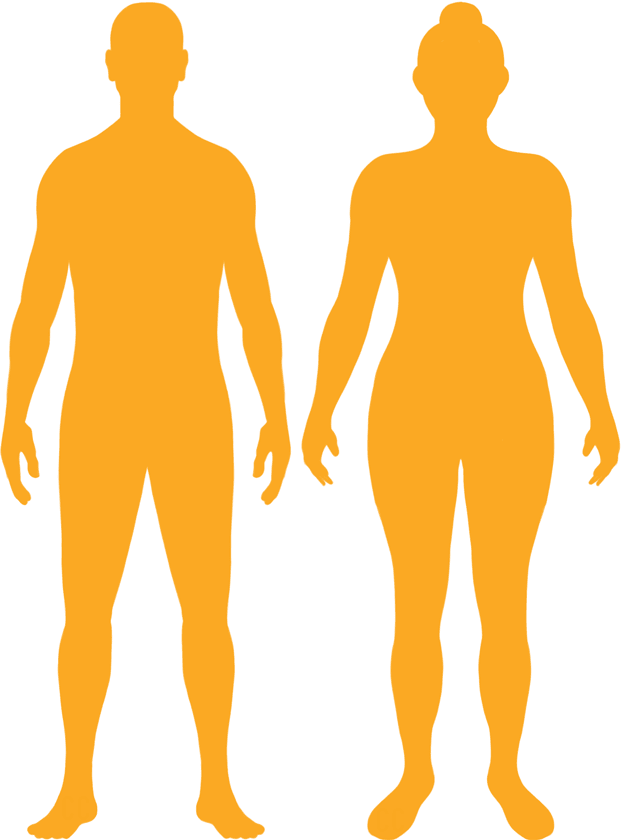# BMI Calculator

Result for 5'11" and 190 lbs.
Change Here.26.5

### Overweight BMI

At 5'11" and 190 Pounds your BMI is 26.5

### BMI Scale

 Under Normal Over Obese
-11 lbs
to reach a
normal BMI classification.
##### Normal BMI Ends:
179.2 lbs
+25 lbs
until you reach an obese BMI classification.
215.1 lbs

### BMI Grading Table2 for Height 5'11"

 Weight Weight Class Below 132.6 lbs Underweight 132.6 lbs - 179.2 lbs Normal Weight 179.2 lbs - 215.1 lbs Overweight 215.1 lbs and Above Obese

### Weight Loss

If you were seeking a normal BMI weight of 179.2lbs, and you lost two pounds a week4, then you could reach a normal BMI in
5.5 Weeks

### Overweight BMI Silhouette### Ideal Weight for a 5 foot 11 Male or Female2

The ideal weight range using the BMI for a male or female with a height of 5'11" is

### Between

132.6 lbs

and
179.2 lbs
How to Calculate BMI3 for 5'11" and 190 lbs
(5 x 12) + 11 = 71
(190 / (71 x 71)) x 703
= 26.496726839913
5 = foot | 11 = inch | 190 = weight lbs

### Conversion

• 5 foot 11 = 71 inches
• 5 foot 11 = 180.34cm
• 190 pounds = 13 stone 8 pounds
• 190 pounds = 86.18 kg
• 190 pounds = 86182.55 grams

### 5'11" and 190 lbs Summary

What is the BMI for a 5'11" and 190 lbs female? 26.5 BMI, Overweight.
What is the BMI for a 5'11" and 190 lbs male? 26.5 BMI, Overweight.
What is the ideal weight for a 5'11" female? Between: 132.6lbs and 179.2lbs
What is the ideal weight for a 5'11" male? Between: 132.6lbs and 179.2lbs
If I am 5ft 11in and weigh 190 lbs, is that a good weight for my height? Under the BMI classification, 190 lbs is classed as being Overweight.